# Injecting modes in bent and angled waveguides#

Here, we illustrate how we can use the ModeSource and ModeMonitor objects to study modes in bent and angled waveguides. This is very useful in simulations such as the waveguide to ring coupling.

:

# standard python imports
import numpy as np
import matplotlib.pyplot as plt
import matplotlib as mpl

# tidy3D import
import tidy3d as td
from tidy3d import web
from tidy3d.plugins.mode import ModeSolver



## Bent waveguide setup#

First, we will study mode injection and decomposition in a microring. We start by defining various simulation parameters, and the structures that enter the simulation. We simulate a silicon ring on a silicon oxide substrate, and the ring is defined using two Cylinders.

:

# Unit length is micron.
wg_height = 0.22
wg_width = 0.9
# Radius of the simulated ring

# Waveguide and substrate materials
mat_wg = td.Medium(permittivity=3.48**2)
mat_sub = td.Medium(permittivity=1.45**2)

# Free-space wavelength (in um) and frequency (in Hz)
lambda0 = 1.55
freq0 = td.C_0 / lambda0
fwidth = freq0 / 10

# Simulation size inside the PML along propagation direction

# Simulation domain size, resolution and total run time
sim_size = [sim_length, 2 * (radius + 1.5), 3]
run_time = 20 / fwidth
grid_spec = td.GridSpec.auto(min_steps_per_wvl=20, wavelength=lambda0)

# Substrate
substrate = td.Structure(
geometry=td.Box(
center=[0, 0, -sim_size],
size=[td.inf, td.inf, 2 * sim_size - wg_height],
),
medium=mat_sub,
)

# The ring is made by two cylinders
cyl1 = td.Structure(
geometry=td.Cylinder(
center=[0, 0, 0],
length=wg_height,
axis=2,
),
medium=td.Medium(),
)
cyl2 = td.Structure(
geometry=td.Cylinder(
center=[0, 0, 0],
length=wg_height,
axis=2,
),
medium=mat_wg,
)



## Running the simulation#

First, we visualize the simulation to make sure we have set up the device correctly. We will use 'absorber' boundaries along the x-direction, because these boundaries work better than PML for structures which are not translationally invariant along the boundary normal direction.

:

# Simulation
sim = td.Simulation(
center=[sim_length / 2 - 0.2, 0, 0],
size=sim_size,
grid_spec=grid_spec,
structures=[substrate, cyl2, cyl1],
sources=[],
monitors=[field_mnt, flux_mnt],
run_time=run_time,
boundary_spec=td.BoundarySpec(
x=td.Boundary.absorber(), y=td.Boundary.pml(), z=td.Boundary.pml()
),
)

fig = plt.figure(figsize=(11, 4))
gs = mpl.gridspec.GridSpec(1, 2, figure=fig, width_ratios=[1, 2])
sim.plot(z=0, ax=ax1)
sim.plot(x=0, ax=ax2)
plt.show()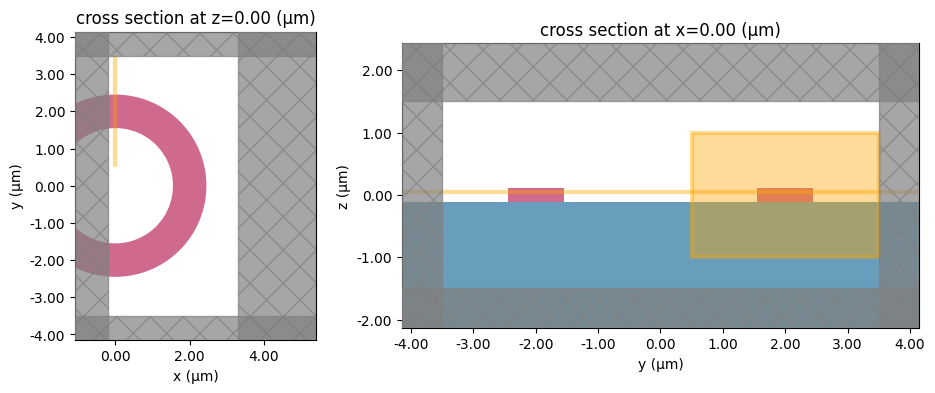Note that Tidy3D is warning us that the simulation does not contain a source. However, since this simulation is used to construct the mode solver and will not be run directly, we can ignore this warning.

Next, we can compute the source modes to make sure that we inject the desired mode. When a bend radius $$R$$ is used, the effective index $$n$$ returned by the solver is such that the field evolves as $$e^{i n k_0 R \phi}$$, with $$\phi$$ the polar angle and $$k_0 = \omega/c$$. This definition is such that in the limit of infinite $$R$$, the effective index approaches that of a straight waveguide with the same cross-section. Based on our discussion and diagram above, we set the bend_axis to 1, and the bend_radius at the position of the source is negative.

:

# Modal source plane
source_plane = td.Box(center=[0, -radius, 0], size=[0, 3, 2])

num_modes = 4
ms = ModeSolver(simulation=sim, plane=source_plane, freqs=[freq0], mode_spec=mode_spec)

modes = ms.solve()
f, axes = plt.subplots(num_modes, 3, tight_layout=True, figsize=(15, 12))
for axe, mode_index in zip(axes, range(num_modes)):
for ax, field_name in zip(axe, ("Ex", "Ey", "Ez")):
ms.plot_field(field_name, "abs", f=freq0, mode_index=mode_index, ax=ax)
plt.show()


           WARNING: Use the remote mode solver with subpixel  mode_solver.py:141
averaging for better accuracy through
'tidy3d.plugins.mode.web.run(...)'.

[14:05:20] WARNING: Mode field at frequency index 0, mode     mode_solver.py:469
index 2 does not decay at the plane boundaries.

           WARNING: Mode field at frequency index 0, mode     mode_solver.py:469
index 3 does not decay at the plane boundaries.Note that the last two of the computed modes are unphysical and for such modes that do not decay to zero at the plane boundary Tidy3D issues warnings. The fundamental mode looks like what we would expect, and we will use that mode for injection. Below, we also define a mode monitor, which is situated radially from the mode source, and so we use a positive value for the bend radius.

:

# Mode source directly exported from the mode solver above
source_time = td.GaussianPulse(freq0=freq0, fwidth=fwidth)
mode_src = ms.to_source(source_time=source_time, mode_index=0, direction="+")

# Mode monitor after one-half round-trip around the ring; NB: positive radius
mode_mnt = td.ModeMonitor(
size=[0, 3, 2],
freqs=[freq0],
name="modes",
)

sim = sim.copy(update=dict(sources=[mode_src]))
sim = sim.copy(update=dict(monitors=[field_mnt, flux_mnt, mode_mnt]))


:

sim_data = web.run(sim, task_name="ring_mode", path="data/sim_data.hdf5", verbose=True)


[14:05:29] Created task 'ring_mode' with task_id                   webapi.py:188
'fdve-e72836d9-ee47-4db7-8e28-be596cf13686v1'.

           View task using web UI at                               webapi.py:190
e72836d9-ee47-4db7-8e28-be596cf13686v1'.

[14:05:35] status = queued                                         webapi.py:361

[14:05:45] status = preprocess                                     webapi.py:355

[14:05:51] Maximum FlexCredit cost: 0.040. Use                     webapi.py:341
'web.real_cost(task_id)' to get the billed FlexCredit
cost after a simulation run.

           starting up solver                                      webapi.py:377

           running solver                                          webapi.py:386

           To cancel the simulation, use 'web.abort(task_id)' or   webapi.py:387
web UI. Terminating the Python script will not stop the
job running on the cloud.

[14:06:06] early shutoff detected, exiting.                        webapi.py:404

           status = postprocess                                    webapi.py:419

[14:06:12] status = success                                        webapi.py:426

[14:06:13] loading SimulationData from data/sim_data.hdf5          webapi.py:590


Finally, we visualize the results and verify that we get very close to unity transmission through the half-circle, and all the power is in the fundamental ring mode.

:

print("Transmission flux:       ", abs(sim_data["flux"].flux.data))
# note: 'backward' mode amplitude
mode_flux = abs(sim_data["modes"].amps.sel(direction="-")) ** 2
print("Flux in first two modes: ", np.array(mode_flux).ravel())

f, (ax1, ax2) = plt.subplots(1, 2, tight_layout=True, figsize=(15, 5))
ax1 = sim_data.plot_field("field", "Ex", z=0.05, f=freq0, val="real", ax=ax1)
ax2 = sim_data.plot_field("field", "E", "abs^2", z=0.05, f=freq0, ax=ax2)
plt.show()


Transmission flux:        [0.9981177]
Flux in first two modes:  [0.99709518 0.00279819]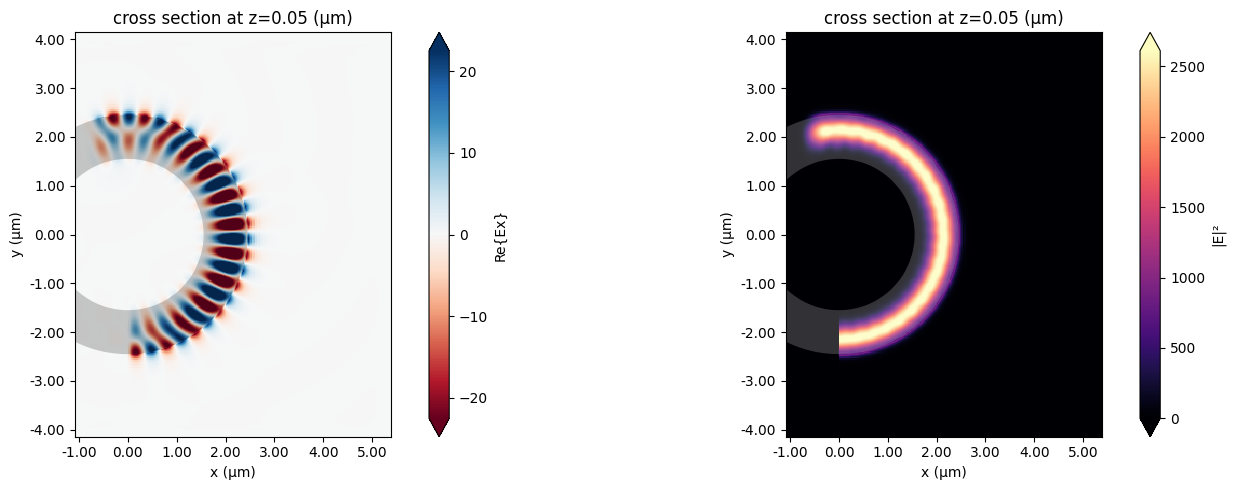## Angled waveguide setup#

Mode objects can also be set to inject and record propagation at a given angle with respect to the axis normal to the mode plane. The angle_theta and angle_phi parameters of ModeSource and ModeMonitor objects define the injection axis as illustrated in the figure below, with respect to the axis normal to the mode plane (x in the figure). Note that angle_theta must be smaller than $$\pi/2$$. To inject in the backward direction, we can still use the direction parameter as also shown in the figure. Similarly, the mode amplitudes computed in mode monitors are defined w.r.t. the forward and backward directions as illustrated.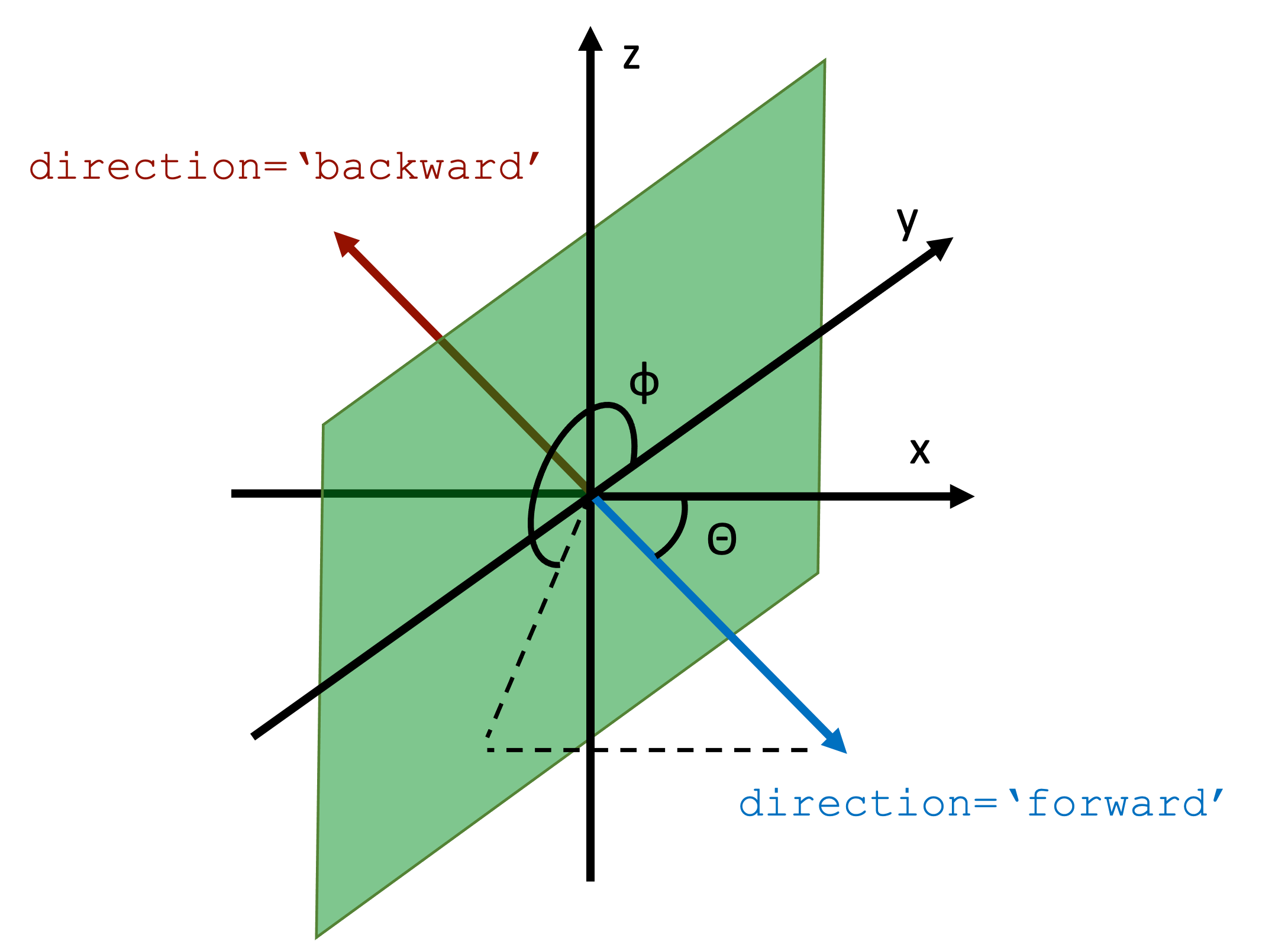:

# Simulation size
sim_length = 3
sim_size = [sim_length, 5, 2]

# Define an angled waveguide as a PolySlab
wg_width = 0.45
theta = np.pi / 4
phi = np.pi

verts_x = np.array([-10, 10, 10, -10])
verts_y = verts_x * np.tan(theta)
verts_y[:2] -= wg_width / 2 / np.cos(theta)
verts_y[2:] += wg_width / 2 / np.cos(theta)
verts_y *= np.cos(phi)  # this only works for phi = 0 or pi
verts = np.stack((verts_x, verts_y), axis=1)
waveguide = td.Structure(
geometry=td.PolySlab(vertices=verts, slab_bounds=(-wg_height / 2, wg_height / 2)),
medium=mat_wg,
)

# Modal source
src_pos = 0
mode_spec = td.ModeSpec(num_modes=2, angle_theta=theta, angle_phi=phi)
msource = td.ModeSource(
center=[src_pos, 0, 0],
size=[0, 3, 2],
source_time=td.GaussianPulse(freq0=freq0, fwidth=fwidth),
direction="+",
mode_spec=mode_spec,
mode_index=0,
)

# Angled modal monitor
mnt_f = td.ModeMonitor(
center=[
sim_length / 2 - 0.5,
(sim_length / 2 - 0.5) * np.tan(theta) * np.cos(phi),
0,
],
size=[0, 3, 2],
freqs=[freq0],
mode_spec=mode_spec,
name="mnt_fwd",
)



We will once again use 'absorber' boundaries along x, since the angled waveguide is not translationally invariant in that direction.

:

# Simulation
sim = td.Simulation(
size=sim_size,
grid_spec=grid_spec,
structures=[waveguide, substrate],
sources=[msource],
monitors=[field_mnt, mnt_f],
run_time=run_time,
boundary_spec=td.BoundarySpec(
x=td.Boundary.absorber(), y=td.Boundary.pml(), z=td.Boundary.pml()
),
)

fig = plt.figure(figsize=(11, 4))
gs = mpl.gridspec.GridSpec(1, 2, figure=fig, width_ratios=[1, 2.2])
sim.plot(z=0, ax=ax1)
sim.plot(x=0, ax=ax2)
plt.show()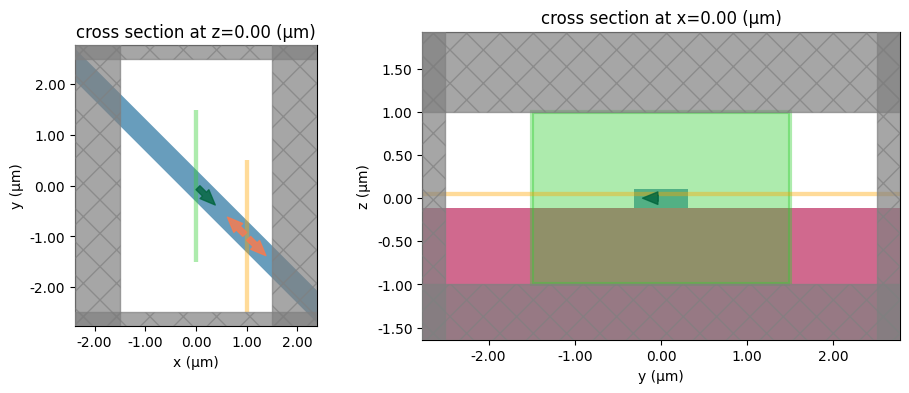Examine the modes. Note that Tidy3D is warning us about the second mode not decaying to zero at the plane boundary, which could mean that this mode is unphysical.

:

ms = ModeSolver(
simulation=sim, plane=msource.geometry, mode_spec=mode_spec, freqs=[freq0]
)
modes = ms.solve()

f, axes = plt.subplots(mode_spec.num_modes, 3, tight_layout=True, figsize=(14, 6))
for axe, mode_index in zip(axes, range(mode_spec.num_modes)):
for ax, field_name in zip(axe, ("Ex", "Ey", "Ez")):
ms.plot_field(field_name, "abs", f=freq0, mode_index=mode_index, ax=ax)
plt.show()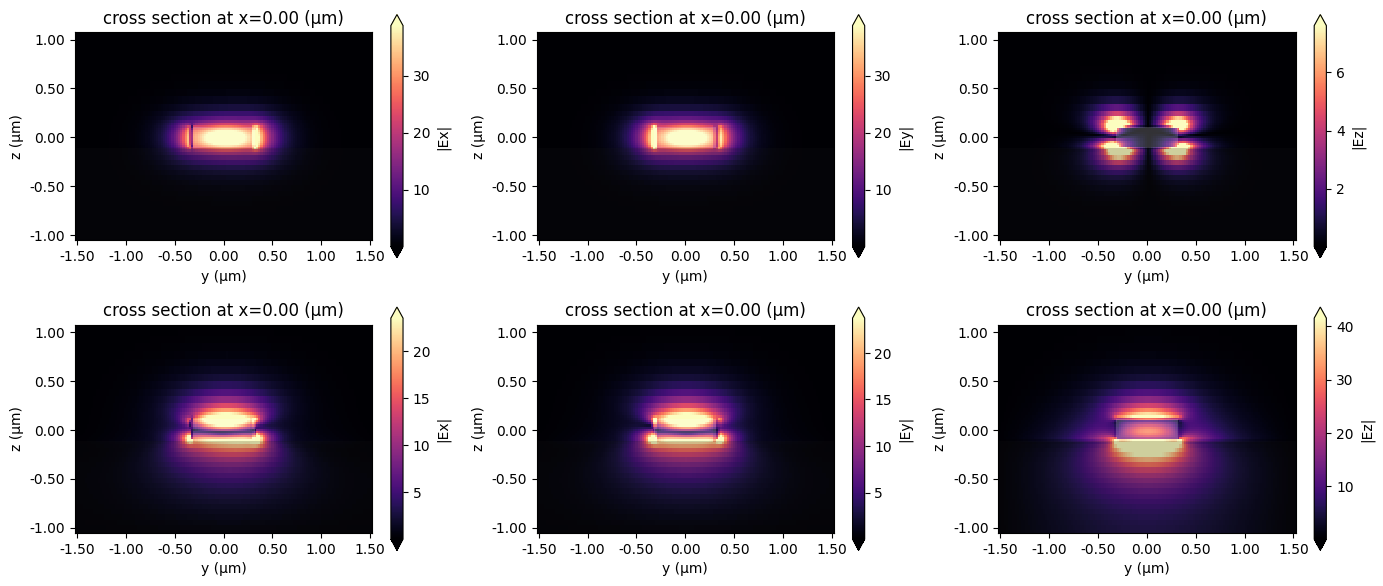Run the simulation and plot the results.

:

sim_data = web.run(
)


[14:06:23] Created task 'angled_waveguide' with task_id            webapi.py:188
'fdve-375b467e-456f-4abf-878c-e0c7e7b3e802v1'.

           View task using web UI at                               webapi.py:190
375b467e-456f-4abf-878c-e0c7e7b3e802v1'.

[14:06:24] status = queued                                         webapi.py:361

[14:06:33] status = preprocess                                     webapi.py:355

[14:06:39] Maximum FlexCredit cost: 0.027. Use                     webapi.py:341
'web.real_cost(task_id)' to get the billed FlexCredit
cost after a simulation run.

           starting up solver                                      webapi.py:377

           running solver                                          webapi.py:386

           To cancel the simulation, use 'web.abort(task_id)' or   webapi.py:387
web UI. Terminating the Python script will not stop the
job running on the cloud.

[14:06:50] early shutoff detected, exiting.                        webapi.py:404

           status = postprocess                                    webapi.py:419

[14:07:03] status = success                                        webapi.py:426

[14:07:04] loading SimulationData from data/sim_data.hdf5          webapi.py:590

:

mode_flux = abs(sim_data["mnt_fwd"].amps.sel(direction="+")) ** 2
print("Flux in first two modes: ", np.array(mode_flux).ravel())

f, (ax1, ax2) = plt.subplots(1, 2, tight_layout=True, figsize=(15, 5))
ax1 = sim_data.plot_field("field", "Ex", z=0.05, f=freq0, val="real", ax=ax1)
ax2 = sim_data.plot_field("field", "E", "abs^2", z=0.05, f=freq0, ax=ax2)
plt.show()


Flux in first two modes:  [9.99327518e-01 6.24054752e-05]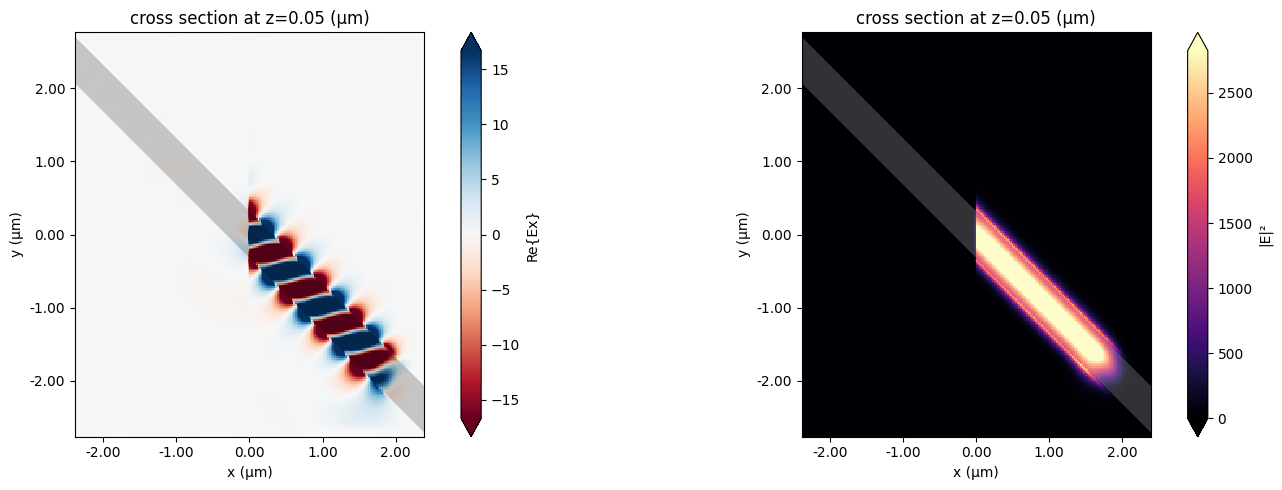## Modes with both a bend and an angle#

We can also compose the two functionalities to inject and record modes in a bent waveguide in which the bend curvature is not in the same plane as the mode plane. This is illustrated below, using the same ring simulation but with a modification of the position of the ModeSource and ModeMonitor.

:

# offset the source and monitor position by 'angle' along the ring
angle = np.pi / 6

# Simulation size for the ring simulation
sim_size = [sim_length, 2 * (radius + 1.5), 3]

# Note: angle_phi = 0, bend_radius = -r
src_angled = td.ModeSource(
size=[0, 3, 2],
source_time=td.GaussianPulse(freq0=freq0, fwidth=fwidth),
direction="+",
mode_spec=td.ModeSpec(
angle_theta=angle,
angle_phi=0,
bend_axis=1,
),
)

# Note: angle_phi = np.pi, bend_radius = r
mnt_angled = td.ModeMonitor(
size=[0, 3, 2],
freqs=[freq0],
mode_spec=td.ModeSpec(
num_modes=2,
angle_theta=angle,
angle_phi=np.pi,
bend_axis=1,
),
name="modes",
)

# Simulation
sim = td.Simulation(
center=[sim_length / 2 - 0.2, 0, 0],
size=sim_size,
grid_spec=grid_spec,
structures=[substrate, cyl2, cyl1],
sources=[src_angled],
monitors=[field_mnt, mnt_angled],
run_time=run_time,
boundary_spec=td.BoundarySpec(
x=td.Boundary.absorber(), y=td.Boundary.pml(), z=td.Boundary.pml()
),
subpixel=True,
)

fig = plt.figure(figsize=(11, 4))
gs = mpl.gridspec.GridSpec(1, 2, figure=fig, width_ratios=[1, 2])
sim.plot(z=0, ax=ax1)
sim.plot(x=0, ax=ax2)
plt.show()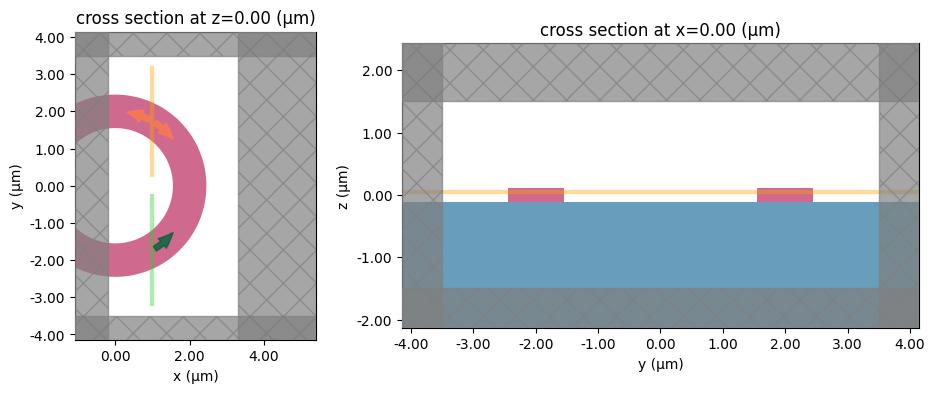:

sim_data = web.run(
)


[14:07:07] Created task 'angled_ring' with task_id                 webapi.py:188
'fdve-3872b199-3ce0-40ae-a23a-cdf96183641ev1'.

           View task using web UI at                               webapi.py:190
3872b199-3ce0-40ae-a23a-cdf96183641ev1'.

[14:07:08] status = queued                                         webapi.py:361

[14:07:16] status = preprocess                                     webapi.py:355

[14:07:21] Maximum FlexCredit cost: 0.040. Use                     webapi.py:341
'web.real_cost(task_id)' to get the billed FlexCredit
cost after a simulation run.

           starting up solver                                      webapi.py:377

           running solver                                          webapi.py:386

           To cancel the simulation, use 'web.abort(task_id)' or   webapi.py:387
web UI. Terminating the Python script will not stop the
job running on the cloud.

[14:07:35] early shutoff detected, exiting.                        webapi.py:404

           status = postprocess                                    webapi.py:419

[14:07:40] status = success                                        webapi.py:426

[14:07:41] loading SimulationData from data/sim_data.hdf5          webapi.py:590

:

mode_flux = abs(sim_data["modes"].amps.sel(direction="-")) ** 2
print("Flux in first two modes: ", np.array(mode_flux).ravel())

f, (ax1, ax2) = plt.subplots(1, 2, tight_layout=True, figsize=(15, 5))
ax1 = sim_data.plot_field("field", "Ex", z=0.05, f=freq0, val="real", ax=ax1)
ax2 = sim_data.plot_field("field", "E", "abs^2", z=0.05, f=freq0, ax=ax2)
plt.show()


Flux in first two modes:  [0.99269782 0.00857491]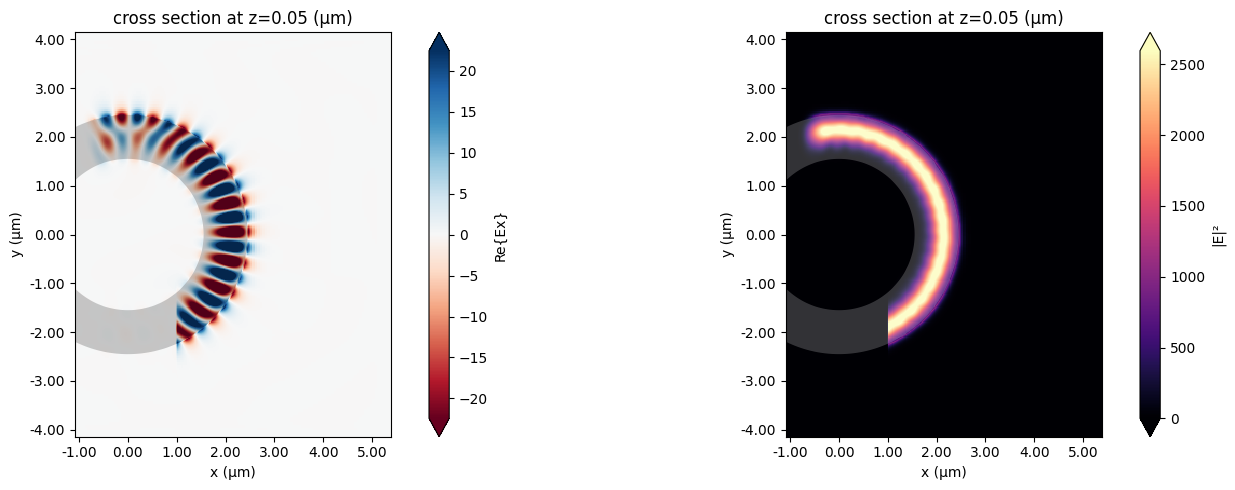For more integrated photonic examples such as the 8-Channel mode and polarization de-multiplexer, the broadband bi-level taper polarization rotator-splitter, and the broadband directional coupler, please visit our examples page.

If you are new to the finite-difference time-domain (FDTD) method, we highly recommend going through our FDTD101 tutorials.

[ ]: